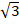Chapter 16.6, Problem 25E

Chapter
Section
Textbook Problem

Find a parametric representation for the surface.25. The part of the cylinder x2 + y2 + z2 = 36 that lies between the planes z = 0 and z = 3To determine

To find: The parametric representation for the part of the sphere x2+y2+z2=36 that lies between the planes z=0 and z=33 .

Explanation

Given data:

The equation of part of the sphere is given as follows.

x2+y2+z2=36x2+y2+z2=62

From the equation of the sphere, the radius of the sphere is 6.

The required part of the sphere lies between the planes z=0 and z=33 .

Formula used:

Write the expression for parametric equations of the sphere with radius r .

x=rsinϕcosθ,y=rsinϕsinθ,z=rcosϕ (1)

Here, the limits of θ and ϕ are written as follows.

ϕlowerϕϕupperθlowerθθupper

Calculation of parametric equations of the sphere:

Substitute 6 for r in equation (1),

x=6sinϕcosθ,y=6sinϕsinθ,z=6cosϕ

Calculation of ϕ limits:

As the required part of the sphere lies between the planes z=0 and z=33 , equate z-parameter to the given values and find the lower and upper limits of ϕ .

Case-i: If z=0

Still sussing out bartleby?

Check out a sample textbook solution.

See a sample solution

The Solution to Your Study Problems

Bartleby provides explanations to thousands of textbook problems written by our experts, many with advanced degrees!

Get Started

Expand each expression in Exercises 122. (4y2)y

Finite Mathematics and Applied Calculus (MindTap Course List)

8. Find all horizontal and vertical asymptotes of the function .

Mathematical Applications for the Management, Life, and Social Sciences

Find the distance between the points. 6. (a, b), (b, a)

Single Variable Calculus: Early Transcendentals

True or False: converges.

Study Guide for Stewart's Multivariable Calculus, 8th